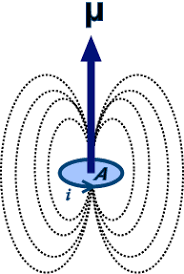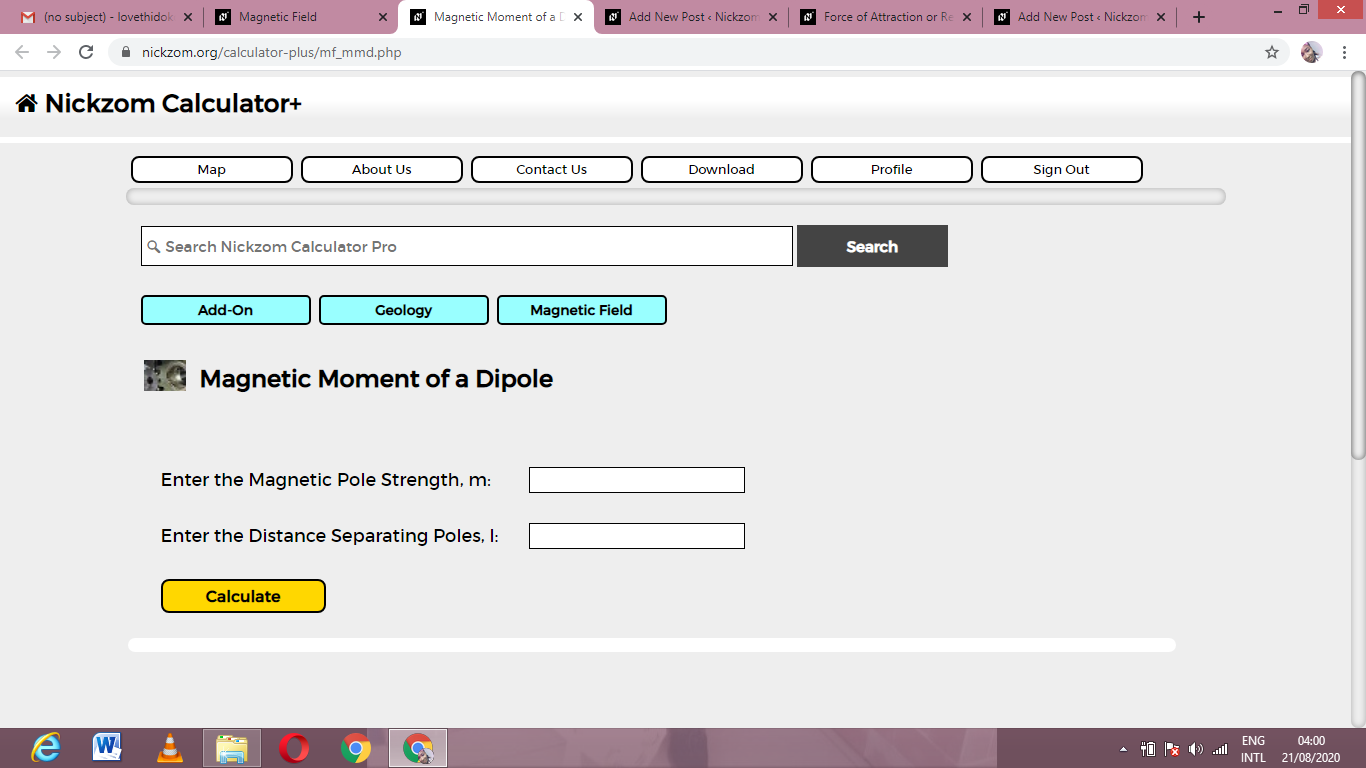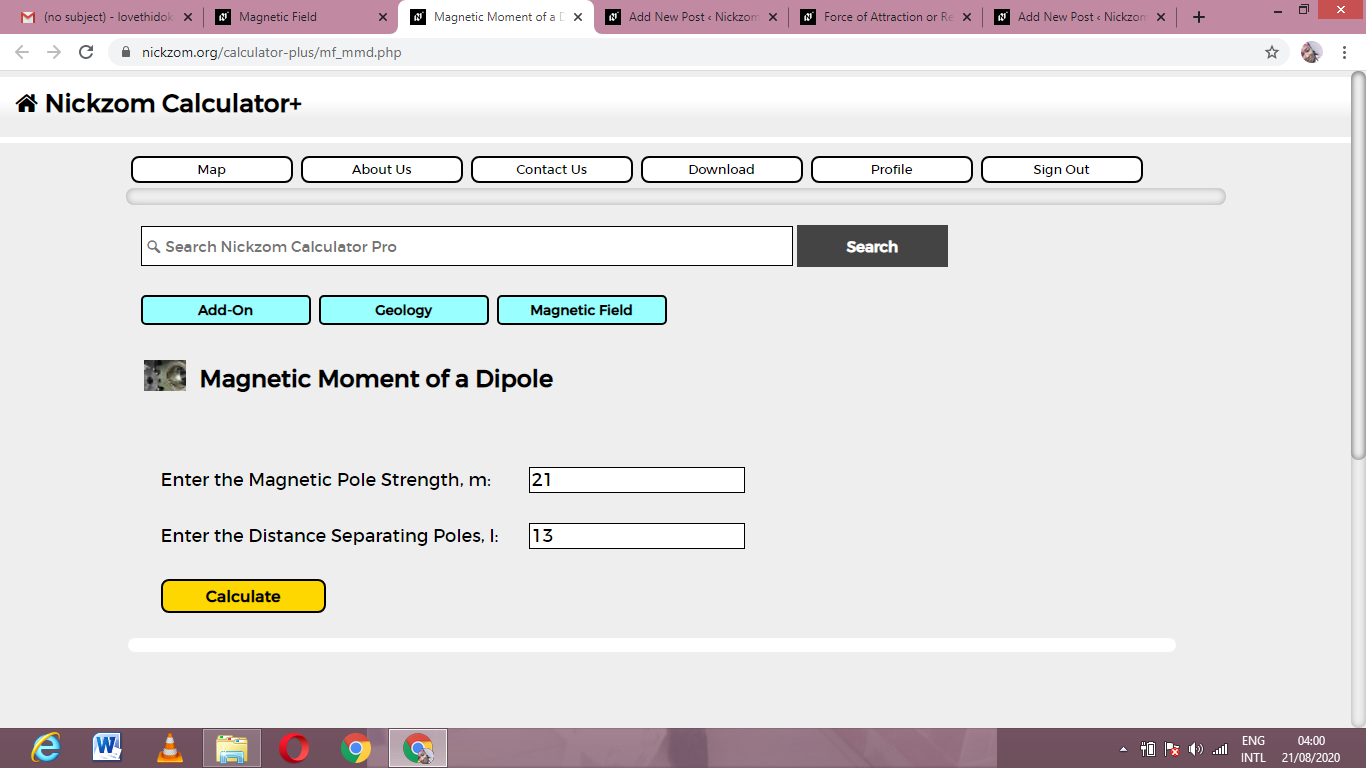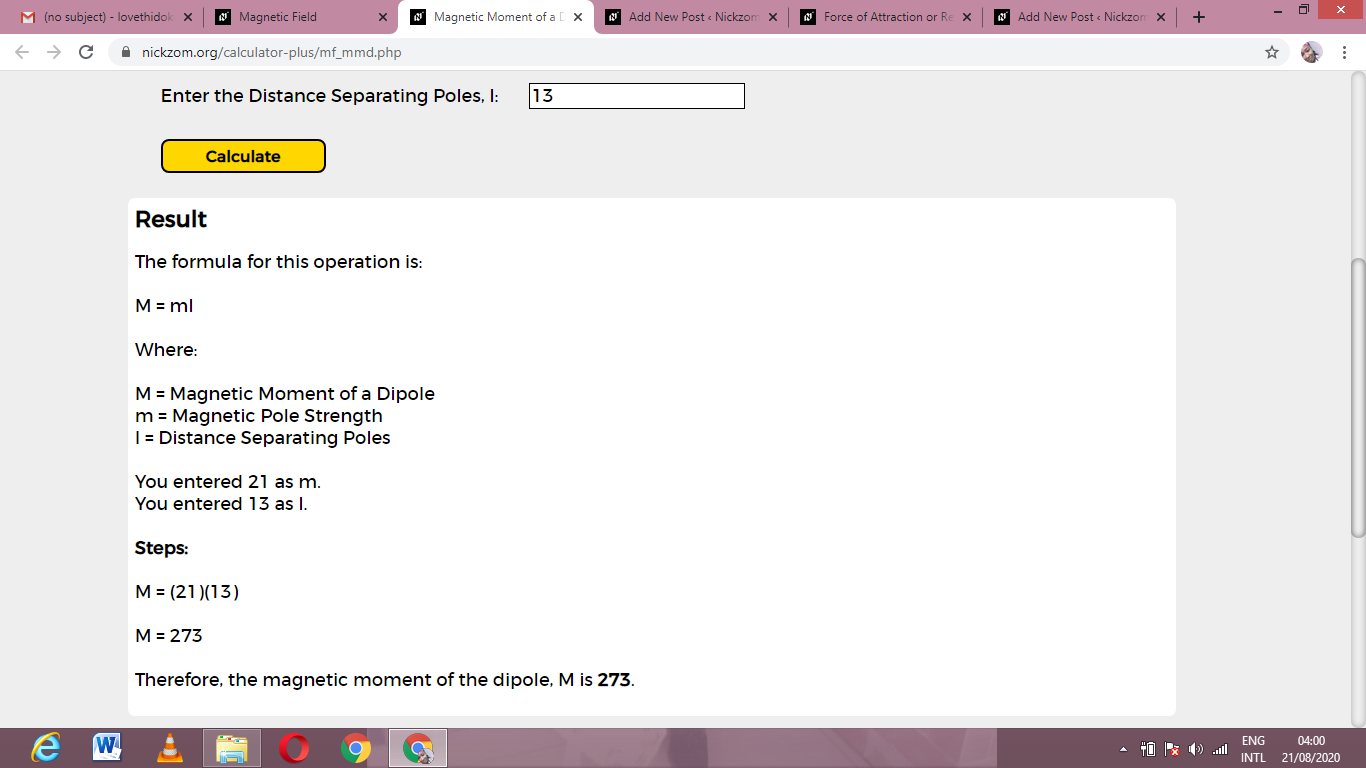# How to Calculate and Solve for Magnetic Moment of a Dipole | Magnetic FieldThe image above represents magnetic moment of a diople.

To compute for magnetic moment of a diople, two essential parameters are needed and these parameters are Magnetic Pole Strength (m) and Distance Seperating Poles (l).

The formula for calculating magnetic moment of a diople:

M = ml

Where;

M = Magnetic Moment of a Diople
m = Magnetic Pole Strength
l = Distance Seperating Poles

Let’s solve an example;
Find the magnetic moment of a diople when the magentic pole strength is 21 and the distance seperating poles is 13.

This implies that;

m = Magnetic Pole Strength = 21
l = Distance Seperating Poles = 13

M = ml
M = 21 x 13
M = 273

Therefore, the magnetic moment of a diople is 273.

Calculating the Magnetic Pole Strength when the Magnetic Moment of a Diople and the Distance Seperating Poles is Given.

m = M / l

Where;

m = Magnetic Pole Strength
M = Magnetic Moment of a Diople
l = Distance Seperating Poles

Let’s solve an example;
Find the magnetic pole strength when the magnetic moment of a diople is 30 and the distance seperating poles is 5.

This implies that;

M = Magnetic Moment of a Diople = 30
l = Distance Seperating Poles = 5

m = M / l
m = 30 / 5
m = 6

Therefore, the magnetic pole strength is 6.

Calculating the Distance Seperating Poles when the Magnetic Moment of a Diople and the Magnetic Pole Strength is Given.

l = M / m

Where;

l = Distance Seperating Poles
M = Magnetic Moment of a Diople
m = Magnetic Pole Strength

Let’s solve an example;
Find the distance seperating poles when the magnetic moment of a diople is 80 and the magnetic pole strength is 4.

This implies that;

M = Magnetic Moment of a Diople = 80
m = Magnetic Pole Strength = 4

l = M / m
l = 80 / 4
l = 20

Therefore, the distance seperating poles is 20.

Nickzom Calculator – The Calculator Encyclopedia is capable of calculating the magnetic moment of a diople.

To get the answer and workings of the magnetic moment of a diople using the Nickzom Calculator – The Calculator Encyclopedia. First, you need to obtain the app.

You can get this app via any of these means:

To get access to the professional version via web, you need to register and subscribe for NGN 2,000 per annum to have utter access to all functionalities.
You can also try the demo version via https://www.nickzom.org/calculator

Apple (Paid) – https://itunes.apple.com/us/app/nickzom-calculator/id1331162702?mt=8
Once, you have obtained the calculator encyclopedia app, proceed to the Calculator Map, then click on Geology under Add-on.Now, Click on Magnetic Field under GeologyNow, Click on Magnetic Moment of a Diople under Magnetic FieldThe screenshot below displays the page or activity to enter your values, to get the answer for the magnetic moment of a diople according to the respective parameters which are the Magnetic Pole Strength (m) and Distance Seperating Poles (l).Now, enter the values appropriately and accordingly for the parameters as required by the Magnetic Pole Strength (m) is 21 and Distance Seperating Poles (l) is 13.Finally, Click on CalculateAs you can see from the screenshot above, Nickzom Calculator– The Calculator Encyclopedia solves for the magnetic moment of a diople and presents the formula, workings and steps too.